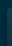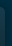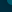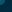# Beatles - Let It Be Acoustic Chords & Tabs## Let It Be Acoustic Chords & Tabs

Version: 2 Type: Chords
`Let It Be Acoustic Chords`

```The Beatles - Let It Be

..INTRO..
Am G F C G F C Dm7 C

C              G                Am          F
When I find myself in times of trouble Mother Mary comes to me
C                   G            F   C Dm7 C
Speaking words of wisdom, let it be
G            Am                   F
And in my hour of darkness she is standing right in front of me
C                   G             F   C Dm7 C
Speaking words of wisdom, let it be

Am         G             F        C
Let it be, let it be, let it be, let it be
C                 G              F   C Dm7 C
Whisper words of wisdom, let it be

C               G              Am            F
And when the broken hearted people living in the world agree
C                  G            F  C Dm7 C
There will be an answer, let it be
C                      G               Am                  F
For though they may be parted there is still a chance that they will see
C                  G            F   C Dm7 C
There will be an answer, let it be

Am         G            F         C
Let it be, let it be, let it be, let it be
C                  G            F   C Dm7 C
There will be an answer, let it be
Am         G            F         C
Let it be, let it be, let it be, let it be
C                   G           F    C Dm7 C
Whisper words of wisdom, let it be
[ Tab from: https://www.guitartabs.cc/tabs/b/beatles/let_it_be_acoustic_crd_ver_2.html ]
SOLO                         ( FAST )             ( FAST )
F C G F C     C G Am F C G F C Dm7 C G Am F C G F C Dm7 C

Am         G             F        C
Let it be, let it be, let it be, let it be
C                 G              F   C Dm7 C
Whisper words of wisdom, let it be

C                 G               Am                 F
And when the night is cloudy there is still a light that shines on me
C              G             F   C Dm7 C
Shine until tomorrow, let it be
C              G               Am          F
I wake up to the sound of music, Mother Mary comes to me
C                   G            F    C Dm7 C
Speaking words of wisdom, let it be

Am         G            F        C
Let it be, let it be, let it be, let it be
C                  G            F   C Dm7 C
There will be an answer, let it be
Am         G            F        C
Let it be, let it be, let it be, let it be
C                  G            F    C Dm7 C
There will be an answer, let it be
Am         G            F        C
Let it be, let it be, let it be, let it be
C                   G           F    C Dm7 C
Whisper words of wisdom, let it be

..END..
F C G F C------

Please leave a comment and tell me what you think.  Thank you.

```